# Tables

#### Introduction to Data Interpretation

Introduction to Data Interpretation

Data Interpretation

What is Data?

Data is the plural of datum, which is basically a Latin noun meaning something given. Today, data is used in English both as a plural noun meaning facts or pieces of information and as a singular mass noun meaning information. Information refers to data being arranged and presented in a systematic or an organized form, so that some useful inferences can be drawn from the same. By data we generally mean quantities, figures, statistics, relating to any event.Data Interpretation

Data Interpretation refers to interpretation or extraction of maximum information from a given set of data or information. At the same time, it is very important that the extracted information is correct and unambiguous.

Unlike Logical Reasoning, wherein you need to solve the puzzle to be able to put forth answers, here you can direct jump straight to the questions, and answer them by analyzing the data and making required Calculations.

For Example

 CLASS 1st 2nd 3rd 4th 5th 6th 7th 8th 9th A 20 22 25 30 33 42 21 35 27 B 33 32 37 44 28 45

Here in the above table, 2 classes of students are under consideration, one of which consists of 9 students and the second one consists only 6 students, whose scores out of 50 marks are mentioned through the columns.

Based on the above, the below mentioned questions can be asked:

Q1) What is the average score of class A?

Q2) What is the average score of class A and B together?

Q3) By what percentage the total marks of class A exceeds that of class B?

Q4) what is the percentage difference between the average marks of classes A and B?

……And many more!

Let’s see how we do that.

Average of N items = Sum/N

Ans 1)

Average score of Class A = (20+22+25+30+33+42+21+35+27)/9 = 28.33333

Ans 2)

Average score of both Class A and B

= (Total marks of class A + Total Marks of class B)/Total Students in both the classes

= (255 + 219)/15

= 31.6

Ans 3)

Total Marks of A = 255

Total Marks of B = 219

Therefore, Difference = 36 Marks

Percentage = (36/219)x100 = 16.4 Percent.

Ans 4)

Do you really think we can calculate this? Well, No!

Even when we know the difference between the 2, we cannot calculate the percentage difference because we don’t know what value to take in the base.

What I mean is,

(36/255)x100

and

(36/219)x100

Both would be correct.

So this was one straight example of how you analyze the data and jump on to solving the questions.

We have a huge variety of such data sets, some relatively easy, and a few difficult to comprehend. But this analysis is what makes you a good manager.

You can understand a hard to solve  DI set to be a Business Challenge that your organization may face in the future.

How to tackle Calculation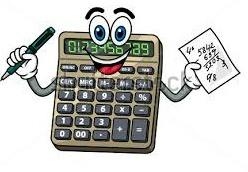The problem that most people fear is the Speed.

You have the Data, you know the way. The only problem is how to calculate faster than the one sitting beside you.

The most frequently asked questions are those related to percentages/average/comparison/ratio etc.

So, I can share a few tricks with which you can add, multiply and find percentage of, in a few seconds.

Let’s say, you want to add the following numbers:

336.456

987.814

126.096

354.348

569.832

Now, this looks like a huge deal!

STEP 1: Hide the part written after decimals, now you are left with only the whole numbers. This Step reduces your Calculations to Half.

STEP 2: Add the numbers normally, you get 2372. (this should not take more than 20 seconds)

STEP 3: Now because you had hidden the Decimals, increase your sum by 3, i.e. make 2372 as 2375.

This is how you can add in half of the original time.

You might just have a doubt that how can we randomly add a number 3.

Let me answer that, it is not random. Of course the fraction part of 5 numbers is definitely less than 5, so we can safely take its total around 3.

Now, the need of adding such huge numbers is generated only when we need to average them out. In that case we will divide the total by 5.

Therefore, the average would be 2375/5 = 475

And if I do the exact addition, it comes out to be 2374.546/5 = 474.909

The difference between our value and the correct value is 0.091. I think this is worth the risk.

So, I expect you to hide the decimals every time you do the adding from now on!

MULTIPLICATION

Multiplying 2 2-digit numbers

For Eg. we multiply 36x87In this step, we multiply

(i) 3 to 7 to get 21

(ii) 8 to 6 to get 48

Add the above 21 and 48 and add the carry 4 to it, we get 73. Write 3 and carry 7 to the third step.In this step, we multiply 3 to 8, we get 24. Now we add the Carry i.e. 7 and add it to 24, we get 31. Write 31 as it is.

Now, I am sure you must be thinking that this is not very short if we compare this method to the conventional one. So for that you can do a small exercise.

Write 5 random 2x2 multiplication questions, Solve all of them first by the conventional method and then by the one I explained above. Note your time on the stop watch, and you will see the difference.

Base Method for Multiplication:

This method of multiplication is lightening quick, but works only when the numbers are near to a common base or a reference number.

Case:1 When both the numbers are greater than the base.

Let’s say we need to multiply 104 by 107.

Step 1: Now, 104 is +4 greater than 100 and 107 is +7 greater than 100 (base here) . Writing it this way :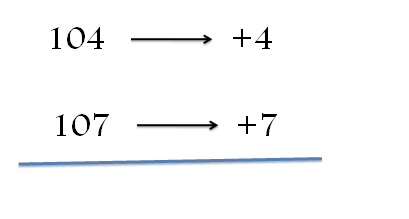Step 2: Add +7 to 104 or  + 4 to 107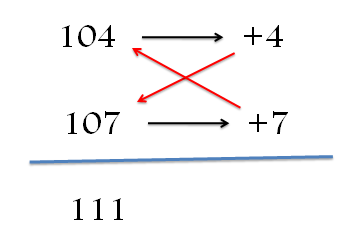Step 3: Multiply this number obtained by base i.e. 100 here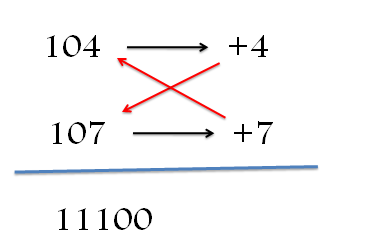Step 4: Multiply +4 with +7 and add the result to the number obtained earlier i.e. 11100 and this will get us the final answer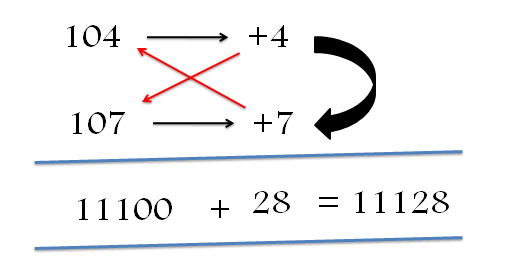Thus, the final answer is 11128

Case2: When both the numbers are less than the base:

Eg. Multiply 196 by 192

Here both the numbers are less than 200 by 4 and 8 respectively. So we take the base as 200.

Step1 :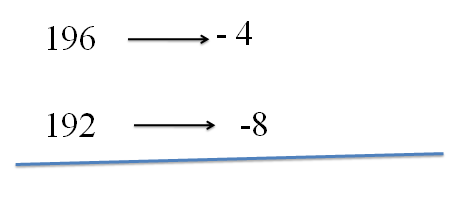Step 2: Add -4 to 192 or -8 to 196 and write the number so obtained.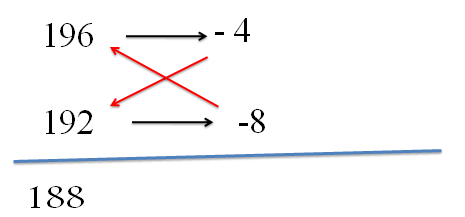Step 3: Multiply the number so obtained by the base i.e. 200 in this case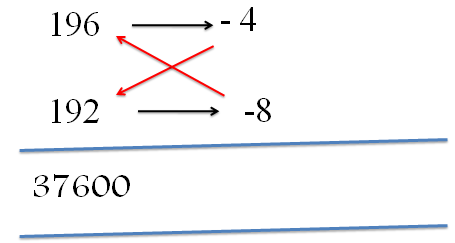Step 4 : Multiply (-4) with (-8) and add the result to the number obtained earlier, i.e. 37600 to get the final answer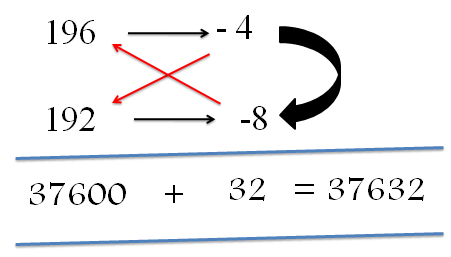Thus, the final answer is 37,632

Case 3: When one number is greater than the base while the other is less than the base.

E.g. Multiply  97 by 105

Here one number is greater than 100 by 5 and one is less than 100 by 3. So here we take 100 as the base.

Step 1: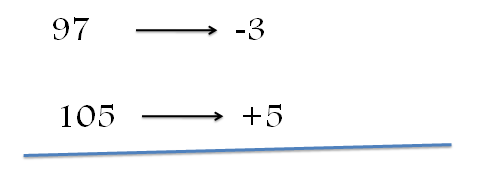Step 2 :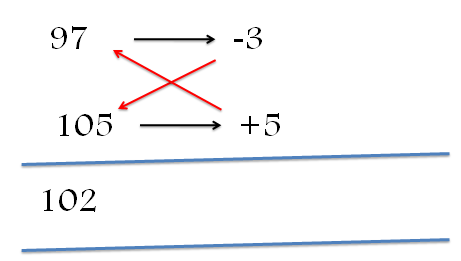Step 3: Multiply the number so obtained by the base i.e. 100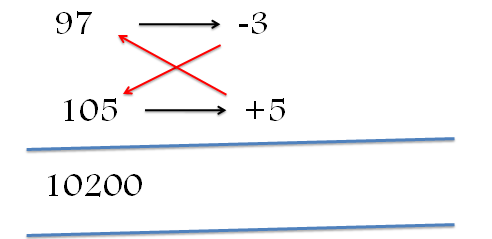Step 4: Multiply (-3) by (+5) and add the result to the number obtained before i.e. 10200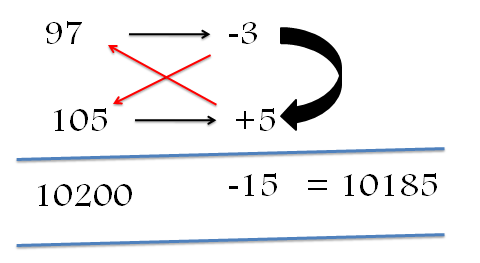Thus, the final answer is 10185.

Try solving some examples and check whether you’ve got the hang of this method.

Multiply the following numbers:

a. 302 * 306

b. 205* 208

c. 47 * 54

d. 99 * 93

e. 246*252

SQUARES

In some topics of Numbers and Arithmetic, you have to calculate squares of huge numbers such as (119)2. Hence, I would like to share some tricks with you, for which you have to fulfill the prerequisite of remembering the squares of numbers from 1 to 30.

 Number Square Number Square Number Square 1 1 11 121 21 441 2 4 12 144 22 484 3 9 13 169 23 529 4 16 14 196 24 576 5 25 15 225 25 625 6 36 16 256 26 676 7 49 17 289 27 729 8 64 18 324 28 784 9 81 19 361 29 841 10 100 20 400 30 900

SQUARING NUMBERS GREATER THAN 100 AND LESS THAN 125:

For eg. (107)2

STEP 1:

Find the difference of this number from 100, it is 7, square of 7=49.

So the Last 2 Digits are 49.

STEP 2:

Add this difference to the number i.e. 107 + 7 = 114

So the first 3 Digits are 114.

The square of the number becomes 11449.

For eg. (119)2

STEP 1

Find the difference of this number from 100, it is 19, square of 19=361.

So the Last 2 digits are 61, and 9 is the carry for 2nd step.

STEP 2

Add this difference to the number itself i.e. 119 + 19 = 138

Add the Carry from the first step i.e. 138 + 3 = 141

So the first 3 digits are 141

The square of the number becomes 14161

SQUARING THE NUMBERS GREATER THAN 75 AND LESS THAN 100

For eg. (94)2

STEP 1:

Find the difference of this number from 100, it is 6, square of 6=36.

So the last 2 digits are 36.

STEP 2:

Subtract this difference from the number itself i.e. 94 – 6 = 88.

So, the first 2 digits are 88.

The square of the number becomes 8836

For eg. (87)2

STEP 1:

Find the difference of this number from 100, it is 13, square of 13 = 169

So the last 2 digits are 69, and 1 is the carry for 2nd step.

STEP 2:

Subtract this difference from the number itself i.e. 87-13 = 74.

Add the carry from the first step i.e. 74+1 = 75

The square of the number becomes 7569

NOTE THAT THE CARRY IS ALWAYS ADDED IN EVERY CASE.

SQUARING THE NUMBERS GREATER THAN 50 AND LESS THAN 75

For eg (53)2

STEP 1:

Find the difference of this number from 50, it is 3, square of 3 = 09

So the last 2 digits are 09.

STEP 2:

Add the difference to 25 i.e. 25 + 3 = 28.

So the first 2 digits become 28

The square of the number is 2809

For eg. (61)2

STEP 1:

Find the difference of this number from 50, it is 11, square of 11 = 121

So the last 2 digits become 21. 1 is the carry for step 2.

STEP 2:

Add the difference to 25 i.e. 25 + 11 = 36.

Add the carry from the 1st step i.e. 36 + 1 = 37.

The first 2 digits are 37

The square of the number is 3721.

SQUARING THE NUMBERS GREATER THAN 25 AND LESS THAN 50

For eg. (42)2

STEP 1:

Find the difference of this number from 50, it is 8, square of 8 = 64

So the last 2 digits become 64.

STEP 2:

Subtract this difference from 25 i.e. 25 – 8 = 17

So the last 2 digits become 17

The square of the number is 1764

For eg (36)2

STEP 1:

Find the difference of this number from 50, it is 14, square of 14 = 196

So 96 become the last 2 digits of the number. 1 is the carry for the 2nd step.

STEP 2:

Subtract this difference from 25 i.e. 25 – 14 = 11.

Add the Carry from the 1st step i.e. 11 + 1 = 12.

The square of the number becomes 1296.

SQUARING NUMBERS ENDING WITH 5

Square of the number ending with 5 always ends with 25. So the last 2 digits are fixed.

For eg. (95)2

STEP 1:

Last 2 digits will be 25

STEP 2:

Multiply 9 by the next number i.e. 10, we get 9x10 = 90.

So the first 2 digits are 90.

The square becomes 9025.

For eg (105)2 = 10 x 11 | 25 = 11025

For eg. (65)2 = 6 x 7 | 25 = 4225

COMPARISON OF FRACTIONS:

So, if you have to compare 3/20 and 5/16, that won’t be a problem at all. Of course the second one is bigger because

Numerator (1) < Numerator (2)

Denominator (1) > Denominator (2)

This makes it obvious.

But if you want to compare (41/87) and (56/121) with the same speed, you can CROSS MULTIPLY.

4187      56121(41 x 121) > (87 x 56)

Hence 41/87 is the bigger fraction.

So, this can be useful in most cases.

You find application of such a method when you have to compare the current value from the previous value, percentage decrease or percentage increase, growth rate or simple average annual growth rate.

Another Method:

We know that  3/5 = 6/10 = 9/15

If we look at the numerator of the second fraction, then it 6 or we have increased the numerator of the fraction (3/5) by +3 and increased the denominator by +5

So, the increase in the numerator as well the denominator are in the ratio of 3:5, i.e. equal to the initial fraction(3/5).Thus, increasing or decreasing the numerator and the denominator in the same ratio will give us a fraction whose magnitude is the same as the initial fraction.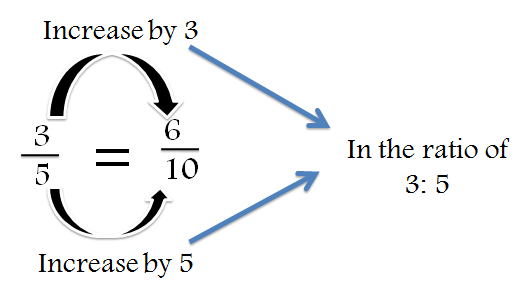Now,this is quite obvious! But ho